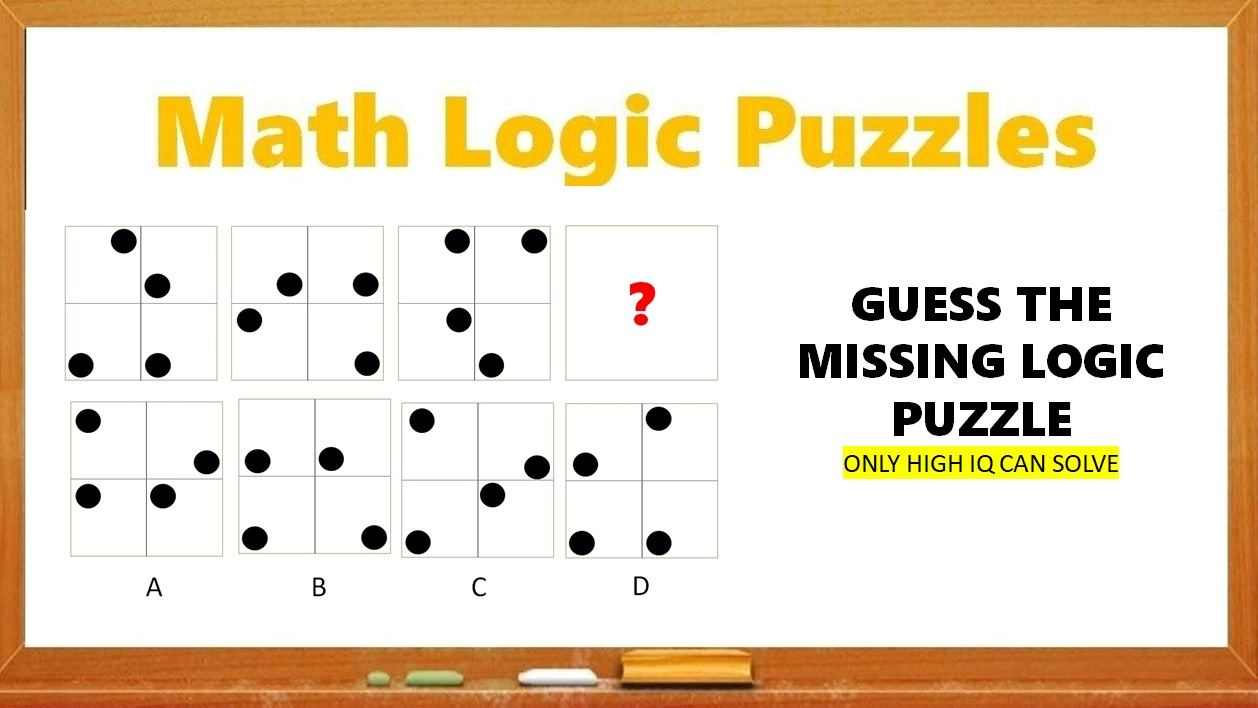# Math Riddles: Hard Logic Puzzles, Only Genius Can Solve in 20 Seconds

Math Riddles Challenge: Can you solve these logic puzzles in 20 seconds each?Math Riddles: Hard Logic Puzzles, Only Genius Can Solve in 20 Seconds

Math Riddles are so challenging, but that makes them worthwhile to solve. Math riddles are logical problems that require strong analytical abilities, high IQ, knowledge of math concepts, and good calculation skills. Math riddles have also been known to make Mathematics enjoyable for kids, students, and even adults to solve. Riddles, puzzles, and brain teasers can develop strategic thinking, logical reasoning, and problem-solving skills.

Today, we bring you another set of math puzzles that are all over social media. These Tricky Logic Math Puzzles have attracted millions of answers but very few got it right.

Can you solve these hard math puzzles? You have 20 seconds each!

## Tricky Math Logic Puzzles Solution

Logic Puzzle #1

Logic Puzzle #2

Logic Puzzle #3

Next, we roll another dice.

Next, we roll the third dice as well.

The dice in place of the question mark matches with option A.

## Tell us in comments: Did you solve these logic puzzles correctly in 20 seconds?

Check out more math puzzles!

Also Read: Math Riddles: Only 1 out 5 Geniuses Can Solve These Math Puzzles

Also Read: Math Riddles: Only 1 out 5 Geniuses Can Solve These Math Puzzles

Also Read: Math Riddles: Solve This Magic Square Puzzle in 20 Seconds

Also Read: Math Riddles: Solve These Hard Logic Puzzles in 20 Seconds Each

... and many more!

Get the latest General Knowledge and Current Affairs from all over India and world for all competitive exams.
खेलें हर किस्म के रोमांच से भरपूर गेम्स सिर्फ़ जागरण प्ले पर